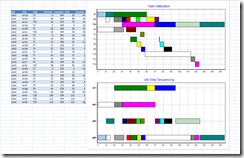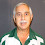## Monday, July 26, 2010

### Scheduling problem formulation

A prototype model was sent to me that was basically a job-shop model. It was formulated with a time index. Unfortunately this model had a large number of time periods, and thus a large model resulted:

* Rows:       4942
* Columns:    10210 (10210 integer, 10210 binary)
* Non-zeros:  137091

The number of time periods needed was estimated as T = sum of processing times. This number is obviously a somewhat generous upper bound and as a result we have a large number of binary variables x[jobstep,timeperiod]. Running some heuristic before solving the MIP can help reducing the estimate for T.

Furthermore, this formulation contained quite a few equations of the form 0 >= 0. The reason was that a particular way was chosen to turn off certain equations:

eq{i in I}: p[i]*(…) >= 0;

where p is a parameter with 0,1 values. Although the LP/MIP pre-solver can take care of these, it is better not to generate those equations at all. I.e. use:

eq{i in I: p[i]=1}: … >= 0;

Even after the presolver removes a lot of rows and columns, the remaining MIP is still quite large:

presolved MIP has 3302 rows, 7339 columns, 124084 non-zeros
7339 integer columns, all of which are binary

With a continuous time formulation (no time index) and better care of generating only equations that are really needed, a much smaller model can be formed:

* Rows:       150
* Columns:    91 (36 integer, 36 binary)
* Non-zeros:  371

In this model T is no longer used to determine the number of variables. However it is used to form some big-M values which are present in “job step i is executed before or after job step j” (i.e. no overlap) constraints.Large scale example:

1.2.